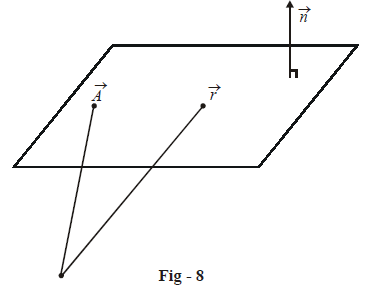# Equations of Three Dimensional Planes

Go back to  'Three Dimensional Geometry'

## Three dimensional plane

In the chapter on Vectors, we have already learnt how to write the equations for a plane, in different forms. In this section, we will extend that discussion and learn how to write the equation of a plane in three dimensional coordinates form.

The general vector equation of a plane is of the form

$$\vec{r}\cdot \vec{n}=l$$; l is a constant

where  $$\vec{r}$$ is the variable vector  $$x\hat{i}+y\hat{j}+z\hat{k}$$  representing any point on the plane, while $$\vec{n}$$  is a fixed vector, say   $$a\hat{i}+b\hat{j}+c\hat{k}$$  which is perpendicular to the plane. Thus, the equation of the plane can be written as

\begin{align}&\qquad\;\left( x\hat{i}+y\hat{j}+z\hat{k} \right)\cdot \left( a\hat{i}+b\hat{j}+c\hat{k} \right)=l \\\\ & \Rightarrow\quad ax+by+cz=l \\\\ & \Rightarrow\quad \boxed{ax + by + cz + d = 0} \quad ;d = - l \\ \end{align}

This is the most general equation of a plane in coordinate form. Note that this equation of the plane contains only three arbitrary constants, for, it can be written as

\begin{align}&\qquad \left( \frac{a}{d} \right)x+\left( \frac{b}{d} \right)y+\left( \frac{c}{d} \right)z+1=0 \\\\ & \Rightarrow\quad {{\lambda }_{1}}x+{{\lambda }_{2}}y+{{\lambda }_{3}}z+1=0 \\\end{align}

Thus, three independent constraints are sufficient to uniquely determine a plane. For example, three non collinear points are sufficient to uniquely determine the plane passing through them.

Example – 9

Write the equation of an arbitrary plane passing through the point  $$A\left( {{x}_{1}},{{y}_{1}},{{z}_{1}} \right).$$

Solution: Let us denote the position vector of A by  $$\vec{A}\,;\,\vec{A}$$  is therefore  $${{x}_{1}}\hat{i}+{{y}_{1}}\hat{j}+{{z}_{1}}\hat{k}.$$  Now, assume that the normal to the plane is $$\vec{n}=a\hat{i}+b\hat{j}+c\hat{k},$$  where a, b, c are variable :Thus, for any variable point   $$\vec{r}=x\hat{i}+y\hat{j}+z\hat{k}$$  on the plane, since  $$\left( \vec{r}-\vec{A} \right)$$  is perpendicular to  $$\vec{n},$$ we have

\begin{align}&\qquad\;\;\left( \vec{r}-\vec{A} \right)\cdot \vec{n}=0 \\\\ & \Rightarrow\quad \left( \left( x-{{x}_{1}} \right)\hat{i}+\left( y-{{y}_{1}} \right)\hat{j}+\left( z-{{z}_{1}} \right)\hat{k} \right)\cdot \left( a\hat{i}+b\hat{j}+c\hat{k} \right)=0 \\\\ & \Rightarrow \quad a\left( x-{{x}_{1}} \right)+b\left( y-{{y}_{1}} \right)+c\left( z-{{z}_{1}} \right)=0\,\,\,\,\,\,\,\,\,\,\,\,\,\,\,\,\,\,\,\,\,\,\,\,\,\,\,\,\,\,\,\,\,\,\,\,\,\,\,\,\,\,\,\,\,\,\,\,\,\,\,\,\,\,\,\ldots \left( 1 \right) \\ \end{align}

This is the required equation of an arbitrary plane through the point $$A({{x}_{1}},{{y}_{1}},{{z}_{1}}).$$

We could have arrived at this equation alternatively as follows: we assume the general equation of a plane which is

$ax+by+cz+d=0\,\,\,\,\,\,\,\,\,\,\,\,\,\,\,\,\,\,\,\,\,\,\,\,\,\,\,\,\,\,\,\,\,\,\,\,\,\,\ldots \left( 2 \right)$

If this passes through $$\left( {{x}_{1}},{{y}_{1}},{{z}_{1}} \right)$$  we have

$a{{x}_{1}}+b{{y}_{1}}+c{{z}_{1}}+d=0\,\,\,\,\,\,\,\,\,\,\,\,\,\,\,\,\,\,\,\,\,\,\,\,\,\,\,\,\,\,\,\,\,\,\,\,\,\,\,\ldots \left( 3 \right)$

By  $$\left( 2 \right)-\left( 3 \right),$$  we arrive at the same equation as in (1).

Learn from the best math teachers and top your exams

• Live one on one classroom and doubt clearing
• Practice worksheets in and after class for conceptual clarity
• Personalized curriculum to keep up with school